# Micro:bit Fishing Game

An accelerometer-controlled fishing game using the BBC Micro:bit.

BeginnerFull instructions provided2 hours333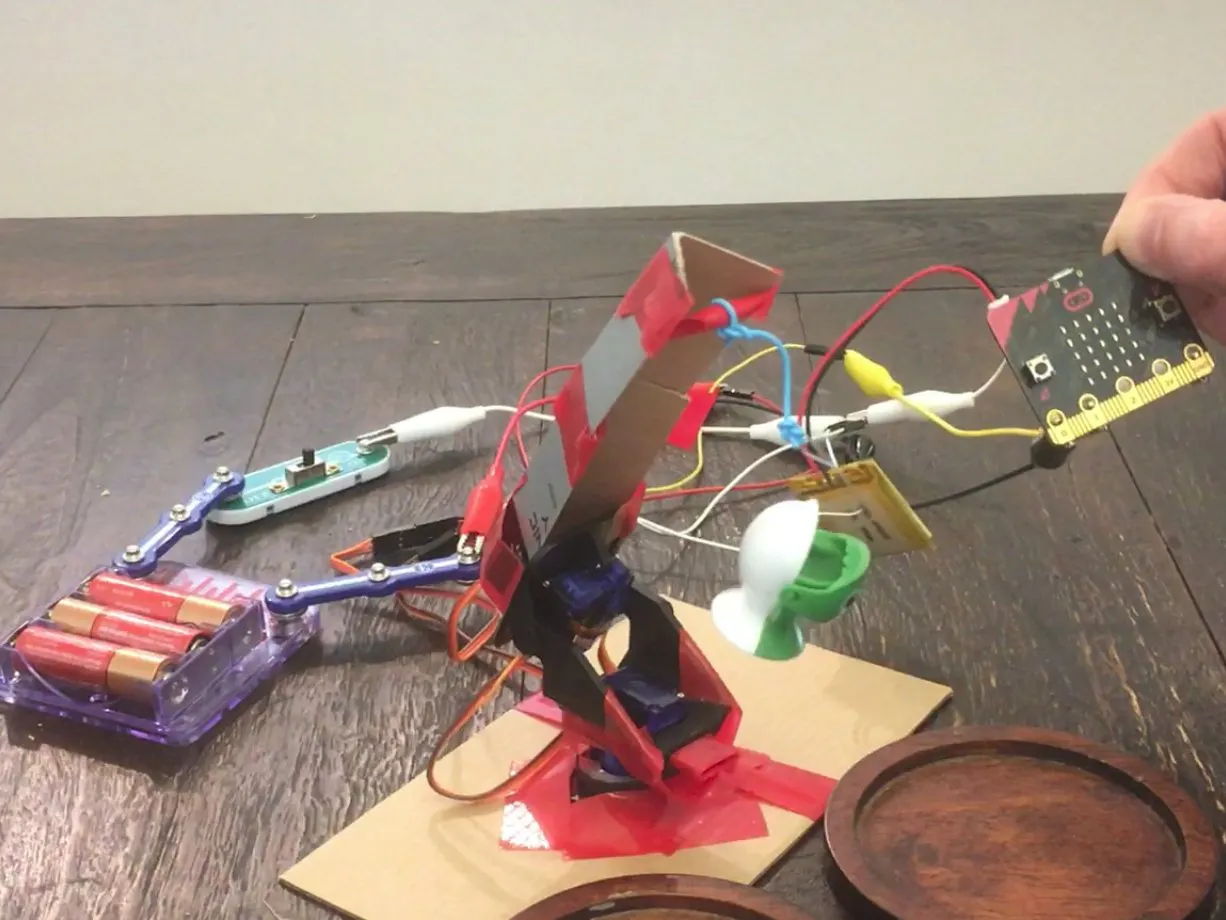## Things used in this project

### Hardware components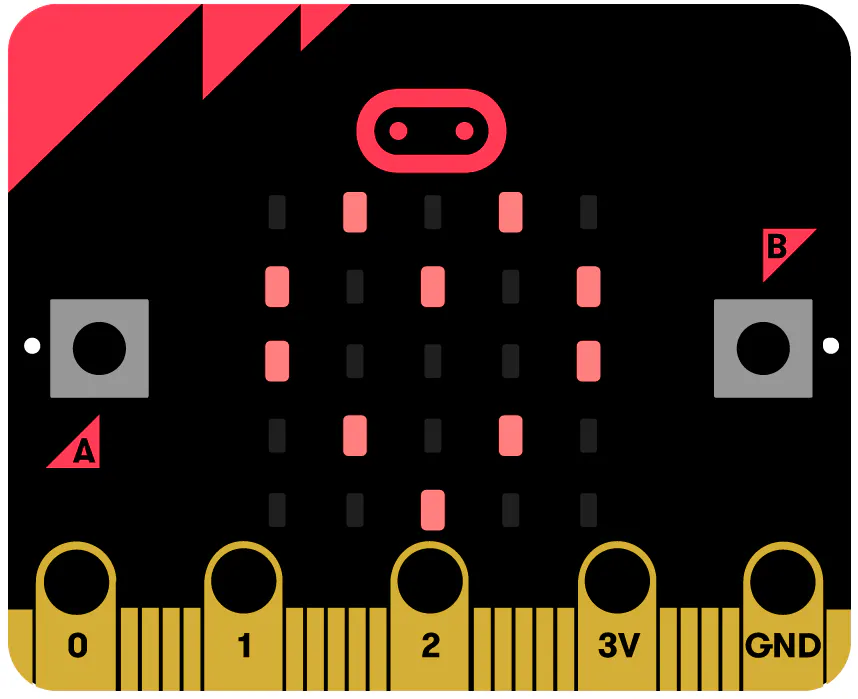BBC micro:bit board
×1
 Adafruit Mini Pan-Tilt Kit - Assembled with Micro Servos
×1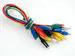Alligator Clips
×1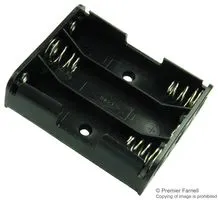Battery Holder, AA x 3
×1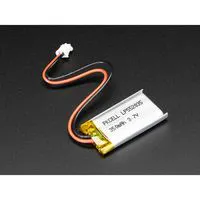Battery, 3.7 V
×1

### Software apps and online servicesMicroPython

## Schematics

### wiring_diagram_LnNj0IrIVA.jpg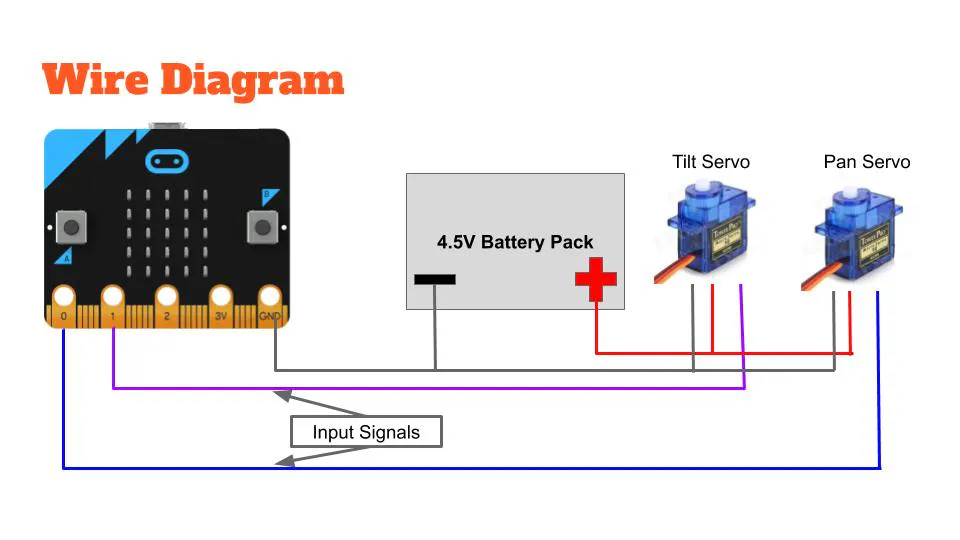## Code

### microbit_servo.py

MicroPython
```# Inspired by: Artronix Jam - War of the Worlds: Rise of the micro:bits Script 1
# Tested with SG90 servo @ 3.3v
# Copy and paste this into the compiler at http://python.microbit.org/editor.html

from microbit import *

class Servo:

"""
A simple class for controlling hobby servos.
Args:
pin (pin0 .. pin3): The pin where servo is connected.
freq (int): The frequency of the signal, in hertz.
min_us (int): The minimum signal length supported by the servo.
max_us (int): The maximum signal length supported by the servo.
angle (int): The angle between minimum and maximum positions.
Usage:
SG90 @ 3.3v servo connected to pin0
= Servo(pin0).write_angle(90)
"""

def __init__(self, pin, freq=50, min_us=600, max_us=2400, angle=180):
self.min_us = min_us
self.max_us = max_us
self.us = 0
self.freq = freq
self.angle = angle
self.analog_period = 0
self.pin = pin
analog_period = round((1/self.freq) * 1000)  # hertz to miliseconds
self.pin.set_analog_period(analog_period)

def write_us(self, us):
us = min(self.max_us, max(self.min_us, us))
duty = round(us * 1024 * self.freq // 1000000)
self.pin.write_analog(duty)
self.pin.write_digital(0)  # turn the pin off

def write_angle(self, degrees=None):
degrees = degrees % 360
total_range = self.max_us - self.min_us
us = self.min_us + total_range * degrees // self.angle
self.write_us(us)

# loop to check accelerometer position then move servos
while True:

# rescale accelerometer x axis to between 0 and 180
rescaled_angle = ((accelerometer.get_x() /12)+90)

# pan servo to the rescaled angle
Servo(pin0).write_angle(rescaled_angle) # write rescaled angle

# rescale accelerometer y axis to between 0 and 180
rescaled_angle_y = ((accelerometer.get_y() /12)+90)

# tilt servo to rescaled angle
Servo(pin1).write_angle(rescaled_angle_y) # write rescaled angle

# provide 0.1 sec pause
sleep(100)
```

## Credits

### jeffreycoen

2 projects • 5 followers
As odd as this sounds, Python got me into hardware.